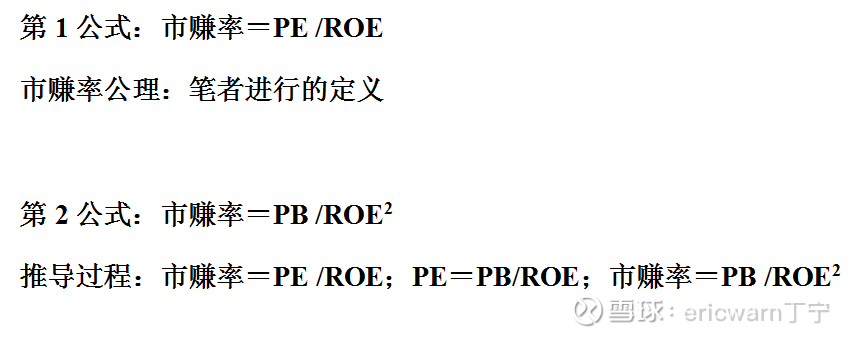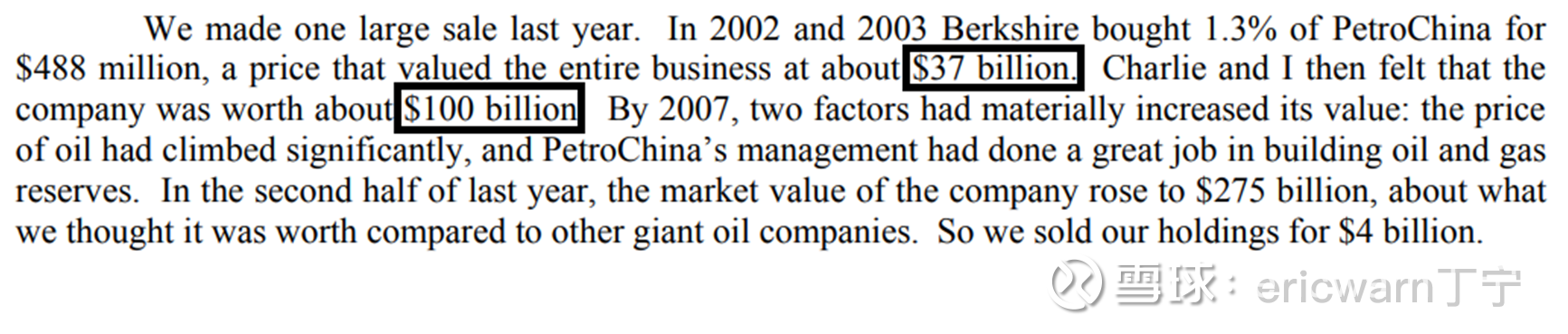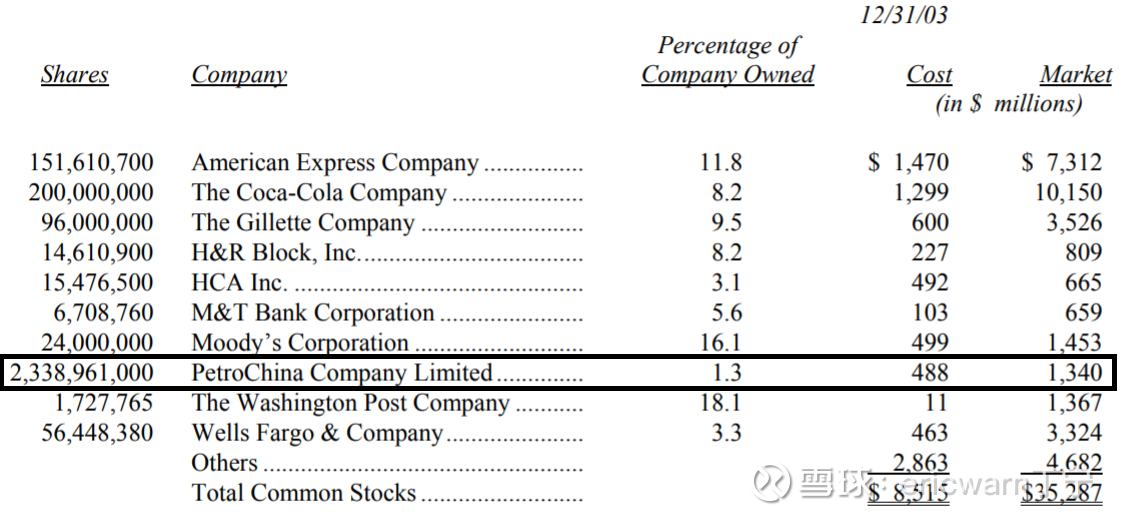# The price-earnings ratio formula is difficult to falsify the first case: PetroChina leaked Buffett’s investment secrets

(PetroChina is the only investment case where Buffett gives an accurate valuation, and the price-to-earning ratio helps you analyze the investment secrets of the stock gods)

Foreword: I recently watched the TV series “Basic Law of Genius” and found that it is not difficult to invent a valuation formula in the investment field. The real difficulty is proving the general correctness of the valuation formula. As the inventor of the P/E ratio, I have recently written three articles on which the P/E formula is difficult to falsify. It can be basically determined that the price-earnings ratio formula is Buffett’s investment secret, and I implore Snowball V to help forward it.

In order to learn from Buffett, the author imitated PEG and invented a valuation parameter called “price-earnings ratio”. The formula is: price-earnings ratio = price-earnings ratio / return on equity (PR = PE / ROE). When the price-earnings ratio is equal to 1PR, it is a reasonable valuation, and if it is greater than 1PR and less than 1PR, it is overvalued and undervalued. Buffett’s classic investment: See’s Candy. The market-to-earnings ratio = 12.5/25 = 0.5PR, which is equivalent to buying at half price. Buffett claims to have changed from “ape” to “human”! In addition, more than 90% of Buffett’s classic investment cases, including Coca-Cola, PetroChina, and Apple, are in line with the P/E valuation system. It should be noted that there is also a derived second formula for the price-earnings ratio (PR=PB/ROE/ROE). When investing in cyclical stocks, the current PB and multi-year average ROE can be substituted into the second formula to calculate the price-earnings ratio valuation of cyclical stocks.Looking back at Buffett’s shareholder letters over the years, only two companies have publicly analyzed the intrinsic value, namely the Washington Post and PetroChina. The former is old and unclear. The latter date is more recent, and the data are detailed. In his 2007 shareholder letter, Buffett stated that the H-shares of PetroChina, which he started to buy in 2002, had a total market value of about \$37 billion at that time. “Charlie and I both felt that the intrinsic value of the company should be about \$100 billion.”It is understood that PetroChina H shares were listed in 2000, so the public financial report can only be found in 1999. During the period from 1999 to 2002, the ROE of PetroChina was 12.83%, 20.23%, 15.65% and 14.81% respectively, and the average ROE for many years was 15.88%. As for Buffett’s investment in 2002, PetroChina’s net assets per share have reached 1.8 yuan.

According to Berkshire’s 2003 financial report, Buffett spent a total of US\$488 million to buy 233,896,000 H shares of PetroChina, with an average price of US\$0.21 per share. At that time, the exchange rate of USD to RMB was about 8.277:1, so Buffett’s cost of holding a position per share was 1.73 yuan, and the corresponding price-to-book ratio valuation was 0.96PB. Using the second formula of price-earnings ratio (PR=PB/ROE/ROE), price-earnings ratio=0.96×100/15.88/15.88=0.38PR.As mentioned earlier, when Buffett invested, the total market value of PetroChina was only US\$37 billion, and the intrinsic value corresponding to the price-earnings ratio can be set as the unknown X. Following this context, list an equation: 0.38/1=37 billion/X. After a simple calculation, X equals \$97.4 billion. As a result, Buffett, in his shareholder letter, stated that its intrinsic value was approximately \$100 billion, rounding up (the English word about).

Last but not least, a closer look at the P/E formula reveals a problem. That is under the premise of constant ROE and 100% retained earnings. Buying and selling companies with different ROEs at 1PR valuation, the higher the ROE, the higher the investment compound interest will be. For a company with an ROE of 30%, the theoretical investment compound interest of the market-to-earning ratio is as high as 30%. For a company with an ROE of 20%, the theoretical investment compound interest of the market-to-earning ratio is only 20%. In this case, why doesn’t Buffett blindly pursue high ROE companies? In fact, high ROE companies are more likely to experience declines in performance and suffer double kills from Davis. Therefore, the market-to-earning ratio gives high ROE companies a higher theoretical investment compound interest, which is actually a kind of risk compensation. It is precisely because companies with high ROE are difficult to maintain for a long time, so Buffett lowered the minimum threshold of ROE to 15%.

Last but not least, the P/E formula is by no means a panacea. But it can go beyond the price-earnings ratio and price-to-book ratio, providing a clearer margin of safety when investing. As for the success of the investment, it depends on the investor’s personal competence circle (selecting outstanding companies), the company’s moat (long-term stable ROE), and even a little luck (Mr. Market completes the valuation repair faster) .

This topic has 125 discussions in Snowball, click to view.
Snowball is an investor’s social network, and smart investors are here.
Click to download Snowball mobile client http://xueqiu.com/xz ]]>